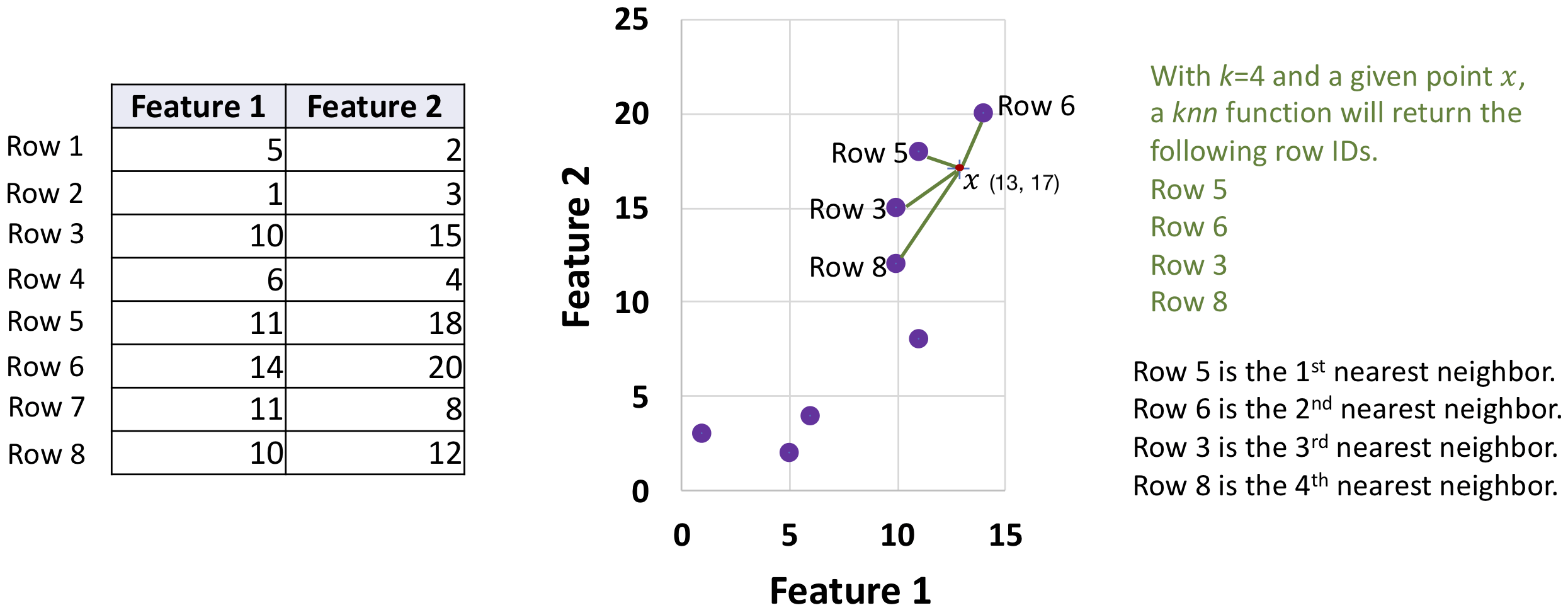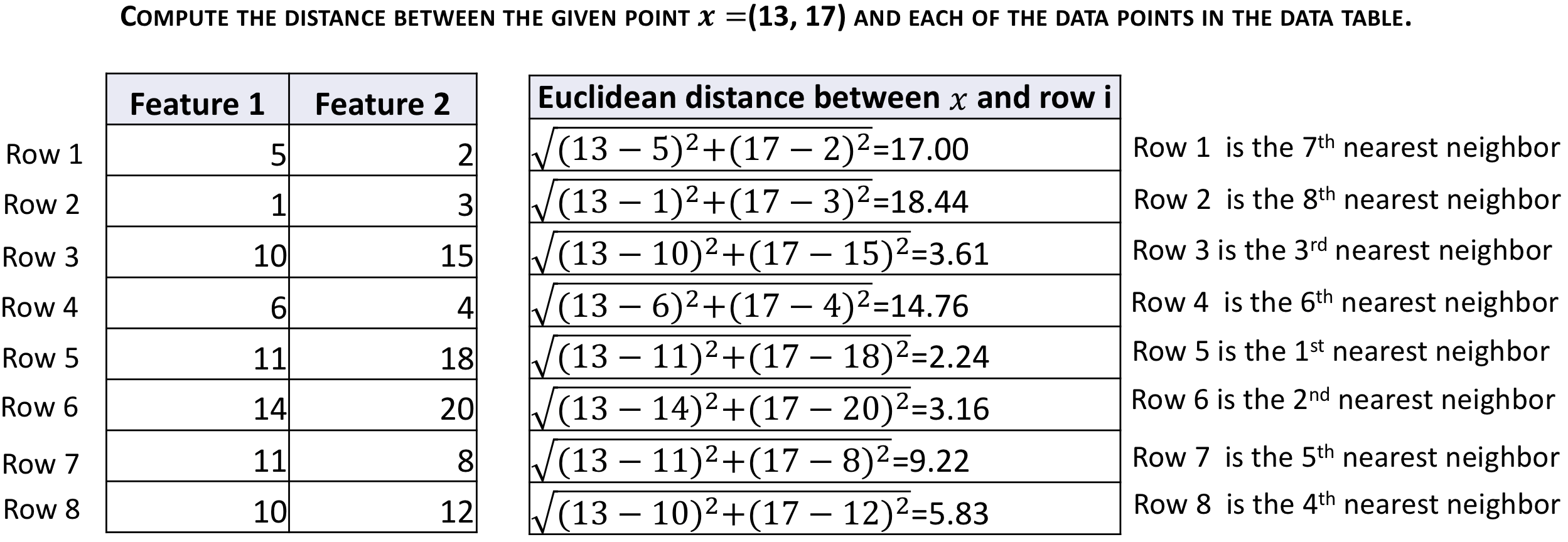• ##### Clustering algorithms
No items in this section

## Introductory Data Science

### Nearest neighbors

Since data forms space and we are already familiar with distance and similarity measures, we can find out points nearby a point. Given a data point, finding several closest points is called the computation of the nearest neighbors. Finding k nearest data points of a given one is know as the knn problem. knn stands for k-nearest neighbors.

## Problem statement for knn

Formally, the knn problem can be written as:

Given a vector$x$ and a data matrix$D$, order all$n$ vectors of$D$ such that$D=\{x_1, x_2, x_3, \ldots, x_n\}$ and$\text{distance}(x, x_i)\leq\text{distance}(x, x_{i+1})$. Return the first$k$ vectors$S=\{x_1, x_2, x_3, \ldots, x_k\}$ where$k\leq n$

For numerical objects, the length of$x$ must be equal to the number of features/dimensions in$D$ to be able to use a distance or similarity measure.

From the programming perspective, knn returns the indices (row serial number) of the top k-nearest neighbors, instead of returning k complete vectors from the data.

The distance function can be any proximity measuring function that we are already familiar with from the previous lessons. You might need a distance measure for certain applications; for other applications, a similarity measure might be alright.

## Example of knn

The table on the right side of the following figure has eight rows (objects) and two columns (features.) A point$x$=(13, 17) is provided. We are attempting to find 4 nearest neighbors of$x$ in the data table. After computing distances between$x$ and each of the points, we found that Row 5 is the nearest point to$x$. The second nearest point is Row 6 of the table. The third nearest point is Row 3. Row 8 contains the fourth nearest neighbor of$x$. A 2D depiction of the points in space and the nearest neighbors are shown in the figure.## The process to compute the nearest neighbors of a given point$x$$x$

To compute the k nearest neighbors of the given point$x$=(13, 17) in the table above, we first computed the Euclidean distance of$x$ with each row of the data. The data point (or row) that has the smallest distance is the first nearest neighbor; the data point with the second smallest distance is the second nearest neighbor. When k=4, we select the rows for the first, second, third, and fourth in ascending order of the computed distance with$x$

The calculations are shown below.For the example above, knn will return an array with content [5, 6, 3, 8], which indicates that Row 5 is the first nearest neighbor, Row 6 is the second nearest neighbor, Row 3 is the third nearest neighbor, and Row 8 is the fourth nearest neighbor.

## Remarks

We used examples of two-dimensional data on this page because it is easy to visualize two-dimensional space. In reality, the data can have any number of dimensions. To use Euclidian distance for knn, the given vector$x$ must have the same number of dimensions.

To find knn from string data, given a string one can compute the edit distance between the given string and each string object in the data.

0 replies